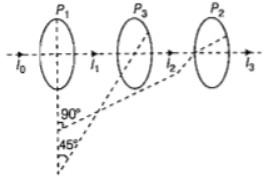# NEET Physics Wave Optics Questions Solved

Watch Physics > Wave Optics VideosNEET - 2017

Two polarids  are placed with their axis perpendicular to each other. Unpolarised light ${I}_{o}$ is incident on ${P}_{1}$. A third polaroid ${P}_{3}$ is kept in between  such that its axis makes an angle ${45}^{°}$ with that of ${P}_{1}$. The intensity of transmitted light through ${P}_{2}$

(a)$\frac{{I}_{o}}{2}$

(b) $\frac{{I}_{o}}{4}$

(c) $\frac{{I}_{o}}{8}$

(d) $\frac{{I}_{o}}{16}$

(c) According to the questionFrom the above diagram, Intensity transmitted through${P}_{3}$

${I}_{2}=\frac{{I}_{o}}{2}{\mathrm{cos}}^{2}{45}^{°}$

Similarly, intensity transmitted through ${P}_{2}$

Difficulty Level:

• 15%
• 34%
• 43%
• 10%
Crack NEET with Online Course - Free Trial (Offer Valid Till September 24, 2019)